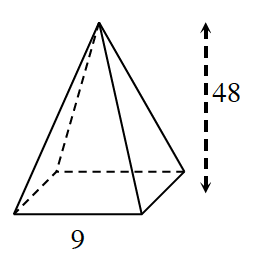### Home > INT2 > Chapter 12 > Lesson 12.2.4 > Problem12-133

12-133.

Multiple Choice: Multiple Choice: What is the volume of the square-based pyramid with base edge $9$ units and height $48$ units?

1. $324 \; \text{units}^3$

1. $1296$ units$^3$

1. $3888$ units$^3$

1. not enough informationRecall the equation of a square-based pyramid: $\frac{1}{3}\left(l\times w\times h\right)$